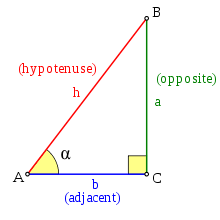SineIn this equation, you need to find the Sine(sin) button on your calculator. This equation helps you find the side Opposite of the angle or the Hypotenuse. For this example, you will do sin(A)=opp/hyp. Lets say you wanted to find the opp side. This will look like sin(A)=opp/hyp , then you multiply the hyp by sin(A), and it will reveal the opposite. If you are tryingto find the Hyp then it is a little different. You will set itup the same like sin(A)=opp/hyp, but then put sin(A) over 1. A represent any angle. You will then cross multiply sin(A) x hyp, and 1 x opp. Set them equal to get sin(A)/hyp = opp, and that is how you use Sine!